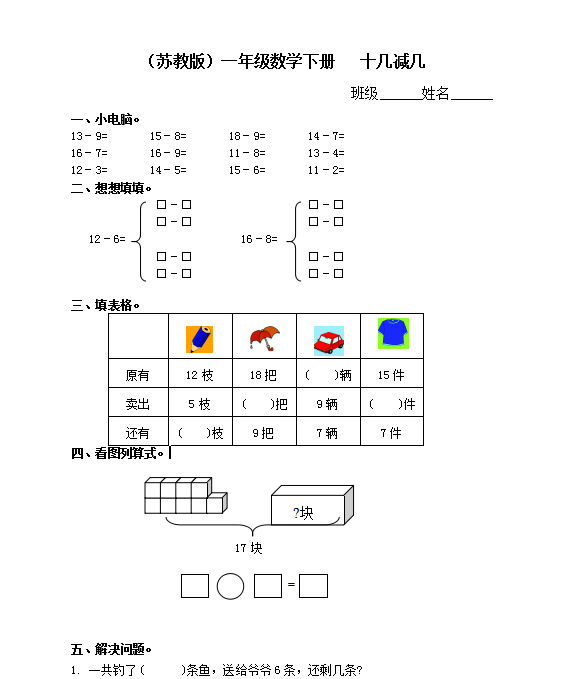（苏教版）小学一年级数学下册 十几减几及答案 2（4页DOC文档）资料下载

（苏教版）小学一年级数学下册   十几减几

13－9=       15－8=       18－9=       14－7=

16－7=       16－9=       11－8=       13－4=

12－3=       14－5=       15－6=       11－2=